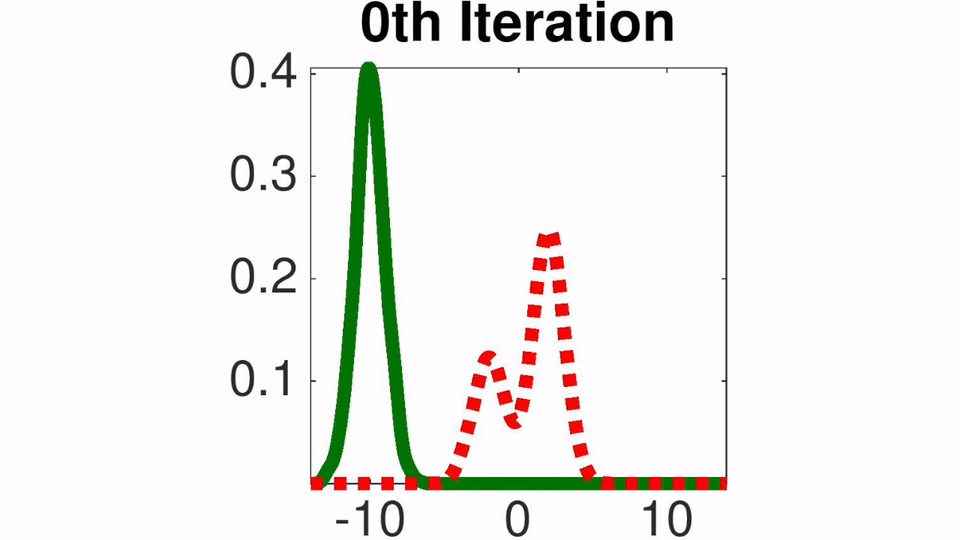code for the paper "Stein Variational Gradient Descent (SVGD): A General Purpose Bayesian Inference Algorithm"
Alternatives To Stein Variational Gradient Descent
Spearmint1,310
6 years ago47Python
Spearmint is a package to perform Bayesian optimization according to the algorithms outlined in the paper: Practical Bayesian Optimization of Machine Learning Algorithms. Jasper Snoek, Hugo Larochelle and Ryan P. Adams. Advances in Neural Information Processing Systems, 2012
Probreg6701a month ago31January 08, 20228mitPython
Python package for point cloud registration using probabilistic model (Coherent Point Drift, GMMReg, SVR, GMMTree, FilterReg, Bayesian CPD)
Bandits472
4 years ago1apache-2.0Jupyter Notebook
Python library for Multi-Armed Bandits
Simple432
6 years ago3agpl-3.0Python
Experimental Global Optimization Algorithm
Btcpredictor269
6 years ago3mitMatlab
Bitcoin price prediction algorithm using bayesian regression techniques
4 years ago1mitPython
code for the paper "Stein Variational Gradient Descent (SVGD): A General Purpose Bayesian Inference Algorithm"
Pilco213
3 years ago12mitPython
Bayesian Reinforcement Learning in Tensorflow
Optimviz119
2 years ago1gpl-3.0MATLAB
Visualize optimization algorithms in MATLAB.
Data_sciences_campaign92
13 hours ago2Jupyter Notebook
【数据科学家系列课程】
Trueskill72
3510 years ago5January 18, 20114mitRuby
a ruby gem that implements the trueskill algorithm
Alternatives To Stein Variational Gradient Descent
Select To Compare

Alternative Project Comparisons

# Stein Variational Gradient Descent (SVGD)

SVGD is a general purpose variational inference algorithm that forms a natural counterpart of gradient descent for optimization. SVGD iteratively transports a set of particles to match with the target distribution, by applying a form of functional gradient descent that minimizes the KL divergence.

The package contains implementation of SVGD in both Matlab and Python language. Demos are also provided to reproduce the results in our paper. The Bayesian neural network example is based on Theano.

## Toy example on 1D Gaussian Mixture

Toy example with 1D Gaussian mixture. The red dashed lines are the target density function and the solid green lines are the densities of the particles at different iterations of our algorithm (estimated using kernel density estimator).## Basic Usage

``````'''
x0: initial particles
dlnprob: returns first order derivative of log probability
n_iter: number of iterations
stepsize: initial learning rate
'''
theta = SVGD().update(x0, dlnprob, n_iter, stepsize)
``````

## Citation

Qiang Liu and Dilin Wang. Stein Variational Gradient Descent (SVGD): A General Purpose Bayesian Inference Algorithm. NIPS, 2016.

## Feedback

Feedback is greatly appreciated. If you have any questions, comments, issues or anything else really, shoot me an email.